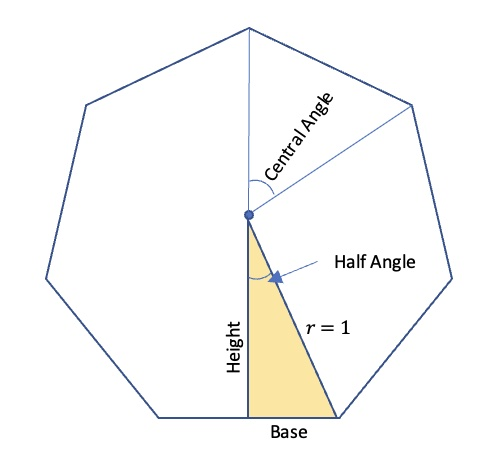# Geometry – Unit Polygons (Part 2)

Welcome Back to our exploration on the areas of Unit Polygons.

So far, we have discovered the areas and perimeters of regular polygons with a Circumradius of one from triangles up to many sides.  Here they are again:

 Sides 3 4 5 6 7 8 9 Area 1.2999 2 2.3776 2.598 2.7365 2.8284 2.8925 Perimeter 5.1962 5.6569 5.8779 6 6.0744 6.1229 6.1564
 10 15 20 30 50 100 150 ∞ 2.9389 3.0505 3.0902 3.1187 3.1333 3.1395 3.1407 3.1416 6.1803 6.2374 6.2574 6.2717 6.2791 6.2827 6.2827 6.2832

Questions:

π is a significant number in mathematics.  What about those other areas?  Such as 2.598 for a hexagon.  Are they analogous to π for a circle?  Could we say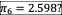Is there a real-world or visual representation of this?

There is some serious thinking in these questions if the students can get engaged in them.  The teacher should use all their superpowers to get them engaged in this discussion.

To begin, we came up with a general equation for the Area of a Unit Polygon as follows: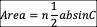This is the trigonometric area of a triangle, which exists in most geometry books and all pre-calculus books.  Where n is the number of sides and C is the central angle (360/n).

This can be simplified for unit polygons to this equation where n is the number of sides.  I let them discover this in groups.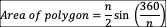### Desmos graph of Areas vs. Number of sides

It is apparent that it converges on 3.14. What is happening below 3?!?!. This is a good example of extraneous solutions.

As expected, the area converges asymptotically on π,  but it gave us more questions such as:

Obviously, the domain of this graph is the set of all integers from 3 to infinity. However, I like to challenge these limits in class.

Questions:

What about unit polygons with a number of sides that is not a whole number?  Can it still be called regular?  How would you draw such a polygon?

What is happening below n=3?  Does this have a significance?  Can they be called polygons if they have less than three sides?

Sometimes in a math class the questions can generate more learning than the answers.  Perhaps the answers should not be pursued but should be allowed to remain in their wondering minds.  Our goal is not always to get correct answers but rather to create strong mathematical minds and mathematical mindsets.  If the questions are all answered, the wonder stops.

Let’s stick with our original question.  What is the significance of the ratio of Perimeter over long diagonal for even unit polygons and long radius + apothem for odd unit polygons.

We compared Perimeter to Area.  For a circle, the ratio is exactly two. For other unit polygons it was not two, so comparing the area of a unit polygon to π didn’t make sense to us.

We graphed this ratio to see if there was an interesting pattern.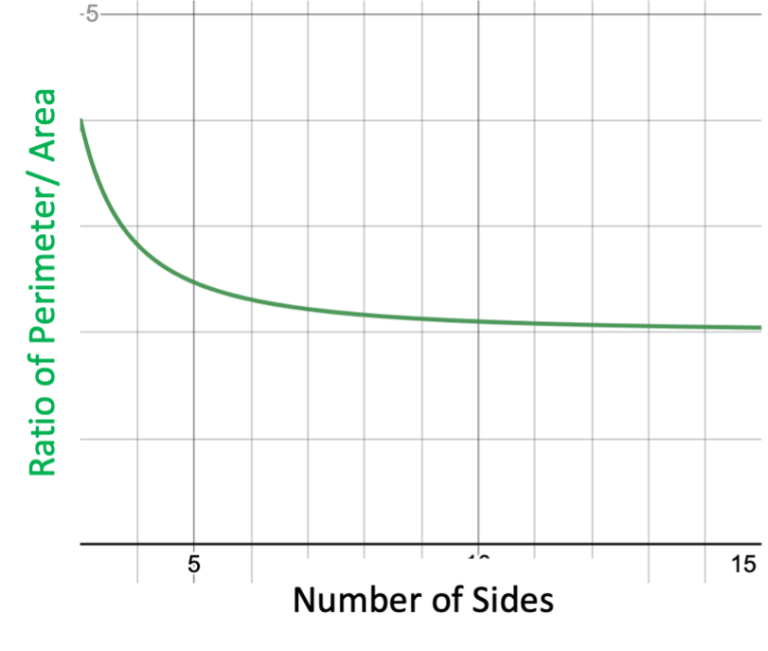It converges on two.  That makes sense because Circumference over Area of a Circle is 2r.  Unfortunately, the π cancels so this does not help us in our efforts to compare the area of a unit polygon to π for a unit circle.

In Part 3, we will continue down the rabbit hole and discuss unit polygons with 1 or 2 sides.  Also, we will look at properties of the apothem.